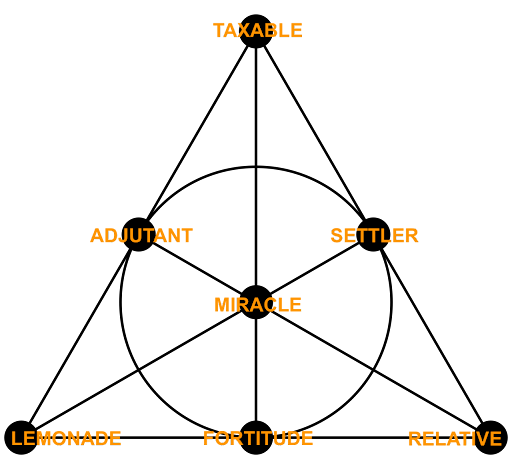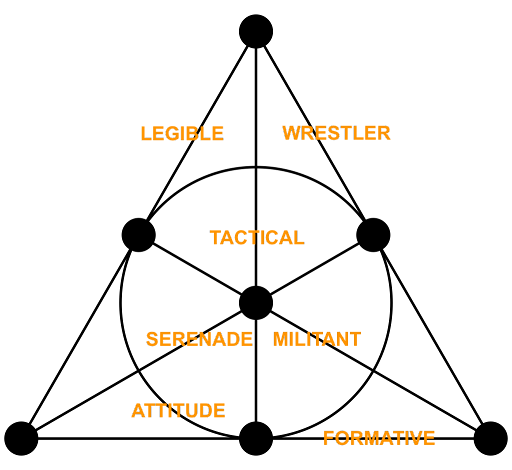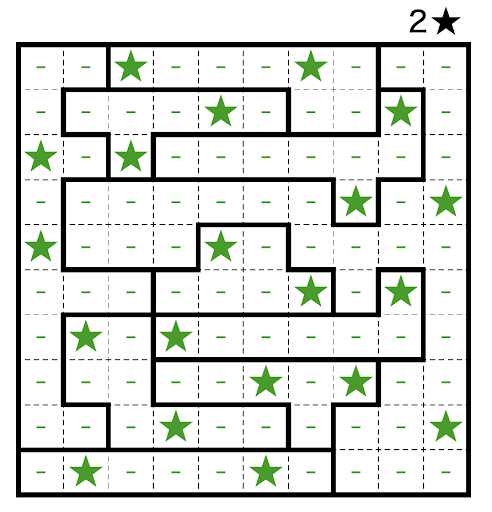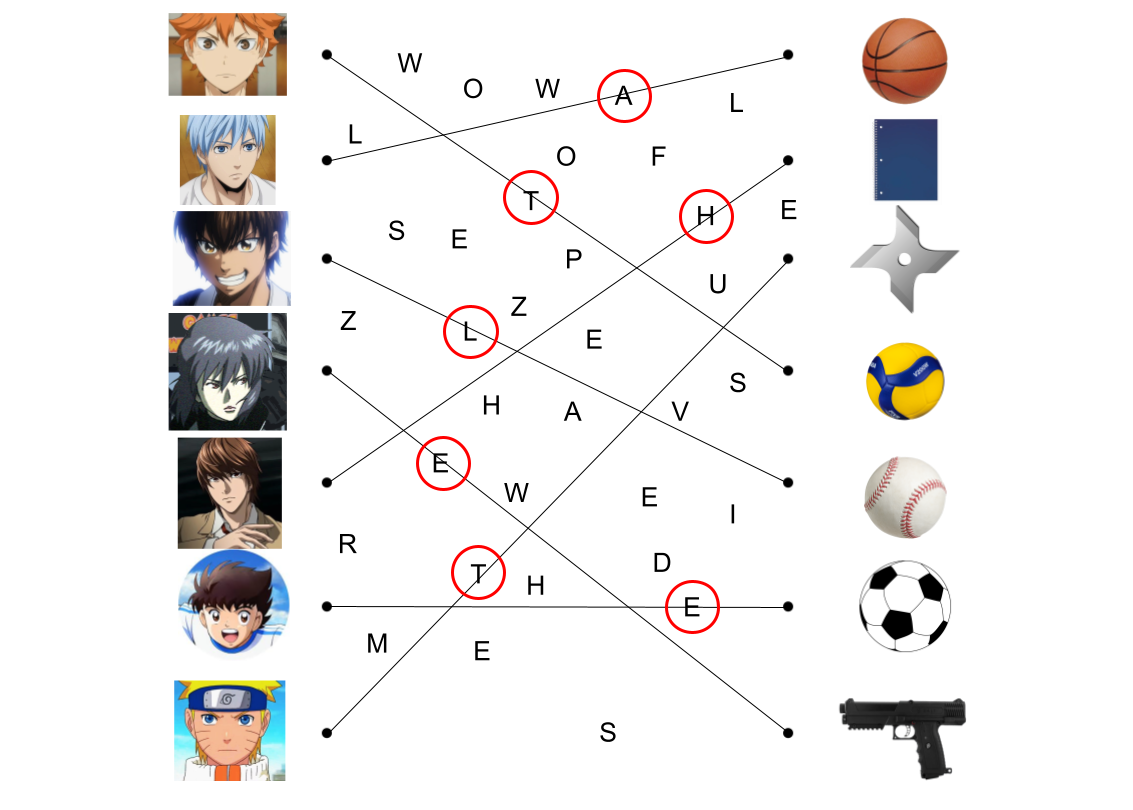# Solution: Fan o' Planes

### Authors:Dan Simon, Benji Nguyen, Shuxin Zhan

The title of this puzzle is cluing a Fano plane, which is a mathematical object with seven points and seven "lines" each with three-point. Each section of this meta has a small image off to the side of three circles connected by a line or circle. These are the "lines" in a standard drawing of a Fano plane. This suggests that each puzzle corresponds to a point, and each schematic represents commonalities between the puzzles and corresponds to a line connecting three of the puzzles. Once the schematics are figured out, we can place the feeder puzzle answers in the Fano plane. We notice that each answer is three¹ syllables. Taking syllables from each answer based on the 1-2-3 in the image next to each section, we get a new word on each "line":This is confirmed by these new words being near-synonyms of the schematics minipuzzle answers (e.g., LEGIBLE on the bird line is a synonym of CLEAR, which is the bird minipuzzle answer). Finally, writing these words in IPA and putting them in the given blanks, we get a phoneme from each word:

• /lɛd͡ʒəbəl/
• /sɛɹəneɪd/
• /mɪlətənt/
• /tæktɪkəl/
• /fɔɹmətɪv/
• /ɹɛsələɹ/
• /ætətud/

These phonemes make /d͡ʒɛtlɔɹd/, which is the IPA for jetlord, the answer and Ruby's username.

### Feeder assignments

Birds
• "True" Friends has a bird as Ruby’s profile image, and in the third location image.
• Make Your Own Puzzlehunt has "something with a bird" as the default logo option, and has a bird as the last hunt reviewer.
• Random Anagramming has a clue "Common city bird" and has "Birds" as one of the ? bank clues.
Brontë sisters:
Grids:
Equals Sign:
• Escape! has an equals sign in an equation on the home screen, as well as in the science lab.
• Tracking Numbers has an equals sign in some clues, along with other mathematical operations.
• Random Anagramming has an equals sign in the flavor (for expert) or several in the puzzle (for casual), and also has a clue with several equations with equals signs.
Triangles:
• Escape! has some triangles (alchemical symbols) on the first screen, as well as in the Knights Templar cipher.
• "True" Friends also has triangles as alchemical symbols.
• Triangular Moves has triangles in its grid.
Anime:
Stick figures:
• Escape! has a stick figure as the gamemaster, in the starting screen and also in each room apart of the Dancing Man cipher.
• Cryptic Images has a stick figure as Emily.
• Make Your Own Puzzlehunt has stick figures as hunt reviewers.

### Minipuzzle solutions

Birds

As clued by "Birds Aren’t Real," remove the bird names and look at what’s left (some of the bird names contain others):

OSTEAGLERIROBIBISINCHCFLJAYAMDOVCARDINALEINCUDUCKCKOOGOLCHHEROWRENNICKIWSWANIKENEGODODOOSECRANSCROWWALOWLLOWEPARFINCHROTAPIGSTORKEONR

OSTRIROBINCHCFLAMDOVEINCUCKOOGOLCHHERONICKIWIKENEGOOSECRANSWALLOWEPARROTAPIGEONR

OSTRICHCFLAMINGOLCHICKENECRANEAR

CLEAR

The remaining letters give the answer CLEAR.

Brontë Sisters

The three relationship webs describe Jane Eyre by Charlotte Brontë, The Tenant of Wildfell Hall by Anne Brontë, and Wuthering Heights by Emily Brontë. The character names give the answer ROMANCE:

1. MRS. REED
2. ARTHUR HUNTINGDON
3. GILBERT MARKHAM
4. BERTHA MASON
5. LINTON HEATHCLIFF
6. CATHY LINTON
Grids

This is a two-star Star Battle. The ultimate solution is:Reading these positions of the letter grid gives us SOLVE WITH BELLIGERENT. The answer is BELLIGERENT.

Equals Sign

These are all famous equations.

Equation name
Standard equation form
Solving for ?
Mass-energy equivalence
E = mc^2
sqrt(E / m) = c
Area of a circle
pi r^2 = A
pi r^2 = A
Area of a rectangle
A = LW
A / W = L
Pythagorean theorem
a^2 + b^2 = c^2
sqrt(a^2 + b^2) = c
Gravitational potential energy
-GMm / R = U
-GMm / R = U
Angular momentum
mvr = L
mvr = L
Newton’s second law
F = ma
F / m = a
Ideal gas law
PV = nRT
PV / nR = T
Electric field strength
F / q = E
F / q = E
Circumference of a circle via diameter
C = pi D
C / pi = D

The question marks give the answer CALCULATED.

Triangles

The two diagrams next to "E.g." have 5 and 7 triangles respectively, which translates to EG under A = 1, Z = 26 (note that some of these triangles overlap or are part of others). The 7 diagrams below have 19, 8, 1, 16, 9, 14, and 7 triangles respectively. Converting via A = 1, Z = 26, we get SHAPING. The answer is SHAPING.

Anime

By connecting the anime characters on the left hand side with their choice of equipment on the right hand side, the lines each intersect with one letter which spells the answer ATHLETE.Stick Figures

This minipuzzle has XKCD panels with text blacked out. We can find the missing text by searching text from the XKCDs.

This forms "One hundred 90 eight title." The title of XKCD 198 is "Perspective". The answer is PERSPECTIVE.

### Authors’ Notes

Answer-choice-wise, this was not as hard to construct as it might seem (we had about 30 answer set options to choose from), with the main difficulty coming from the runtime of option-finding code being roughly 15 minutes.

For the schematics connecting the puzzles, we originally thought about requiring each feeder have three "parts" from each of the seven types (for example, a grid, an image, and a list of clues), but decided this might stymie the creativity of feeders in this round. The schematics we eventually settled on were elements that were easy to write into a puzzle and that we wouldn't mind seeing three times in one round. We sifted through puzzles from older hunts to prove to ourselves that the constraints would be reasonable. We're grateful to all of the writers for this round for working within these constraints.

The original version of this puzzle had no schematic images and no hint at commonalities in the flavor text; the commonalities were solely supposed to come from the minipuzzles. However, testsolving teams were still able to solve the meta by noticing the answers were three¹ syllables and mixing. We felt strongly about wanting the puzzle commonalities to be an important part of the solve path, so the flavor text was edited to aggressively suggest doing this. In the end, this meta probably ended up with more information than required for teams to solve it, but test solving for this puzzle would require a test solve of the whole round, which was impractical given the timeline, so we decided to stay on the side of the puzzle being too easy for teams than vice versa.

1: There was great debate within the team whether SETTLER was 2 or 3 syllables.